0
2137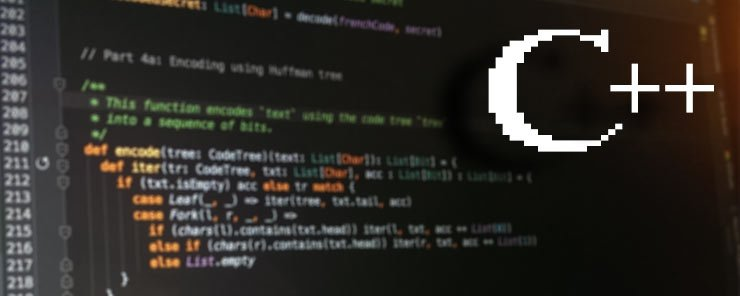Continuing with Strings. In this article, we are going to look at more examples on using the string class. Have a nice reading.

### Initializing a string Object using another string Object

Besides the two forms we learned in the last article, a string object can also be initialized by assigning another string object to it. So if:

`string x = "Hello World";`

The following will assign x to another string object y:

`string y = x;`

### String Concatenation

The string class has overloaded the plus + operator to concatenate string objects.

Example
The following program will append a string to the end of another.

```#include <conio.h>
#include <iostream>
using namespace std;
int main()
{
string str1 = "Have a nice day";
string name = "Mohamed";
str1 = str1 + ", ";
str1 += name;
cout << str1;
getch();
return 0;
}
```

When executed, the program should display the following output: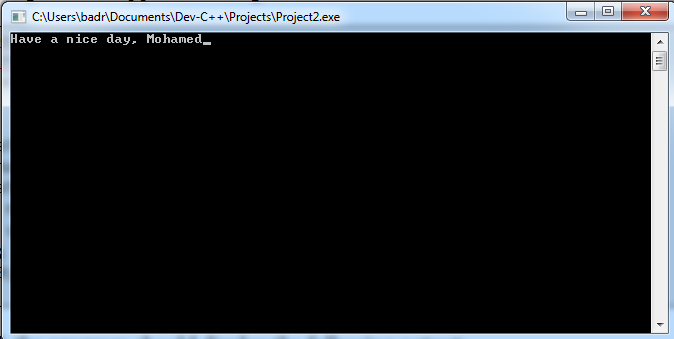Now, let’s explain:

• The statement:
`str1 = str1 + ", ";`

appends a comma and white space to the string object str1.

• The statement:
`str1 += name;`

also appends a string to the end of another. It has the same effect of writing

`str1 = str1 + name;`

### Reading Input for string Objects

The getline() function could be used to read one or more lines of input. Among many overloaded forms of syntax available, the function has the following two commonly-used syntaxes:

```getline(cin, STRINGOBJ);
getline(cin, STRINGOBJ, DELIMITER_CHAR);
```

Where:
cin is the standard input stream object.
STRINGOBJ is the name of the string object to assign the input to.
DELIMITER_CHAR specifies the character which when encountered, will stop the function from reading input.

Example
This example illustrates the use of two forms mentioned above for the getline() function. Read the following code, and copy it into your Dev-C++ IDE:

```#include <iostream>
#include <conio.h>
using namespace std;
int main()
{
cout << "Enter your Name: ";
getline(cin, name);
<< "\nName: " << name
getch();
return 0;
}
```

When executed, the program should behave like this: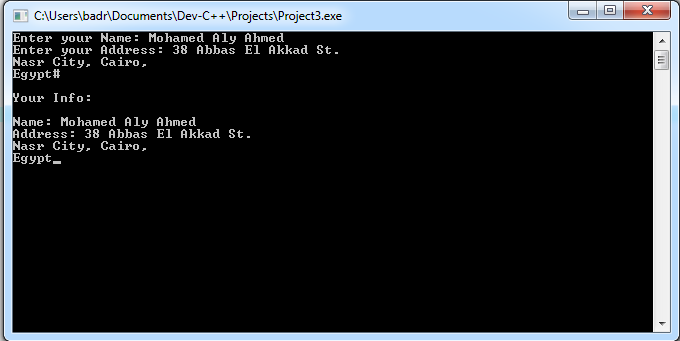As you can see, the statement getline(cin, name); reads a single line of input. On the other hand, the statement getline(cin, address, ‘#’); will continue to read input (that may span several lines) until it encounters the character #.

### Obtaining the Length of the string Object

The length() method returns the number of characters in a string object.

So, if:

`string name="Sherif";`

and

`int len = name.length();`

The variable len will have a value of 6.

### The clear() Method

As its name implies, the clear() method erases the contents of a string object, leaving an empty string behind.

Again, if:

`string name="Sherif";`

the statement

`name.clear();`

will clear the name object.### The erase() Method

The erase() member function removes part of a string object. The part to remove is specified by the starting character position and the number of characters to remove.

Example
The following program illustrates the use of the erase() function.

```#include <iostream>
#include <conio.h>
using namespace std;
int main()
{
string name="Alessandro Del Piero";
cout << name << '\t' << name.length() << endl;
name.erase(0,4);
cout << name << '\t' << name.length();
getch();
return 0;
}
```

When executed, the program will print the following output: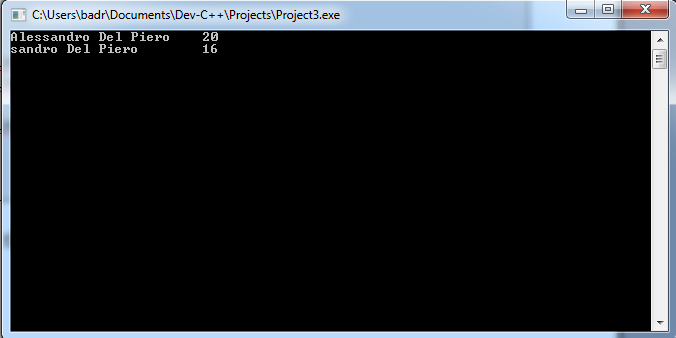The function call:

`name.erase(0,4);`

will erase four characters starting from the first (at position 0).

### The replace() Function

This function replaces a part of the string (identified by starting position and length) by another string, or fills its place by a number of consecutive copies of a character.

Example
The following example illustrates how the replace() function works.

```#include <iostream>
#include <conio.h>
using namespace std;
int main() {
string player("Alessandro Del Piero");
player.replace(11,9, "Nesta");
cout << player;
getch();
return 0;
}
```

When executed, the program will replace the last name of my favorite player by “Nesta”.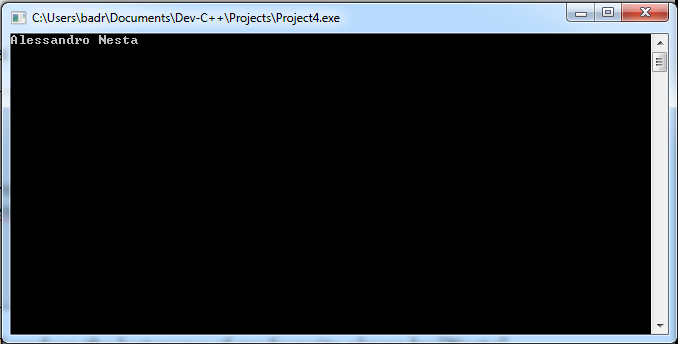### Retrieving a Substring of string Object

The substr() function returns a substring of a string object.

Syntax

`substr(POS, LENGTH);`

Example

Given:

`string player("Alessandro Del Piero");`

The following statement returns a substring of six characters length, starting from the fifth character:

`cout << player.substr(4,6);`

Summary